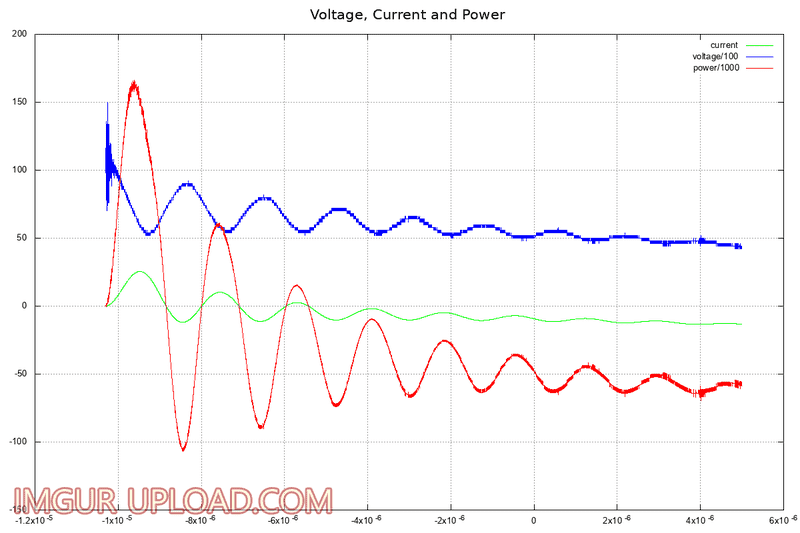# How to calculate the energy of an arbitrary pulse

Dear Sirs,

I need to calculate the energy of the below pulse, as I'm new to dealing with AC I have some doubts on how to calculate it? I think I remember that in order to obtain energy one has to integrate the power, but I'm not sure.

Also I'm not sure how to deal with the calculation of average power as it is arbitrary AC?ps. the power trace is calculated not measured.

#### Attachments

anorlunda
Staff Emeritus
Yes, just integrate voltage times current.

But you may want just the DC component, or the average AC at the fundamental frequency. If so, you must be more specific.

Last edited:
•Abimbola1987 and sophiecentaur
CWatters
Homework Helper
Gold Member
+1

It's the same as it would be for a sin wave AC, it's just that the integral (area under the curve) is easier to work out for a sin wave.

If really stuck with the integral you can even use squared paper to work it out!

•sophiecentaur
Dear anorlunda and CWatters,

Thank you very much for your reply. I do have the data for the wave forms so I have written a small Python script to do the numerical integration.

For the purpose of my understanding; if the wave had a pure sine and you did numerical integration on that, it would equal zero as the negative areas would cancel out the positive, which would imply that the energy is zero? I which case my logic would dictate that the wave had to be offset in some way or have to be calculated with absolute values of y? The same conceptual pondering goes for average power, power is still power even if it's negative?

CWatters
Homework Helper
Gold Member
Yes the absolute value. Aka rectification.

•Abimbola1987
Dear CWatters,

Thank you very much for clearing my uncertainty.

I wish you all the best.
Abimbola

anorlunda
Staff Emeritus
For the purpose of my understanding; if the wave had a pure sine and you did numerical integration on that, it would equal zero as the negative areas would cancel out the positive,

Which wave?

If V and I are both sin waves and in-phase with each other, then when they are +, V*I is +. When they are both -, V*I is + again. So the average of V is zero, the average of I is zero but the average of V*I is not zero.

Which wave?

I meant an imaginary sine wave e.g. the voltage of 220V AC

If V and I are both sin waves and in-phase with each other, then when they are +, V*I is +. When they are both -, V*I is + again. So the average of V is zero, the average of I is zero but the average of V*I is not zero.

Yes, I appreciate that, it seems logical.

Now that I have your attention, could I ask another question regarding integration of Rogowski coil output? Or should I create another post?

anorlunda
Staff Emeritus
Now that I have your attention, could I ask another question regarding integration of Rogowski coil output? Or should I create another post?

A new thread would be better.